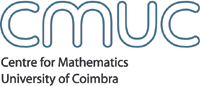Algebra and CombinatoricsMembersSeminarsEventsPublicationsdetails .: download Author(s) Olga Azenhas; Ricardo Mamede; TitleKeys associated with the symmetric group S4 and matrix realizations of pairs of tableaux AbstractWe consider the problem of a matrix realization, over a local principal ideal domain with prime p, for the pair (T,K(\sigma)), where K(\sigma) is a key associated with a permutation of S4, and T is a skew-tableau with the same evaluation as K(\sigma). It has been shown in  that there exists a matrix realization for (T,K(\sigma)), \sigma ∈ St, only if the word of T is in the Knuth class of K(\sigma). The Knuth class of K(\sigma), \sigma ∈ St, contains the shuffles of all their columns, and when the word of T is such a shuffle, the necessary condition for the existence of a matrix realization has also been proved to be sufficient . When  \sigma ∈ St, t ≤ 3, the Knuth class of K(\sigma) is the set of all shuffles of their columns together, but in general this is not the case. For \sigma ∈ S4, we have equality except for the permutations 1423, 1432, 4123 and 4132. In these cases, the Knuth class of any associated key may be described, using shuffling operations, by adding the single word 431421 to the set of the columns of that key. On the other hand, by an injection of the plactic class of a key associated with S4 into S6, we recover the property of describing the elements of a Knuth class by shuffling the columns of the unique tableau in the Knuth class. Additionally the frank words with four columns are explicitly characterized. For any \sigma ∈ S4, an explicit matrix construction for the pair (T,K(\sigma)) is exhibited, when the word of T is in the Knuth class of K(\sigma). The relationship between the matrix and the combinatorial settings is achieved through a variation of the R-S-K correspondence by attaching to a skew-tableau a pair of tableaux of conjugate shapes. Moreover this correspondence exhibits the duality between words congruent with a key and frank words. Preprint seriesPré-publicações do Departamento de Matemática da Universidade de Coimbra Issue06-05 Year2006 CMUC Apartado 3008, 3001 - 454 Coimbra, Portugal T:+351 239 791 150 F:+351 239 793 069 cmuc@mat.uc.pt - developed by Flor de Utopia